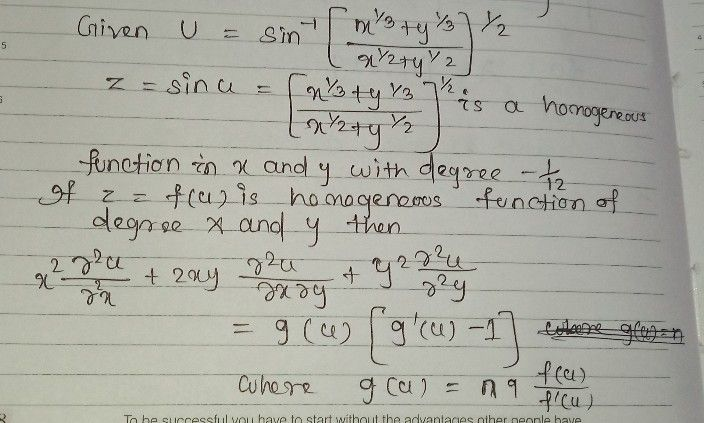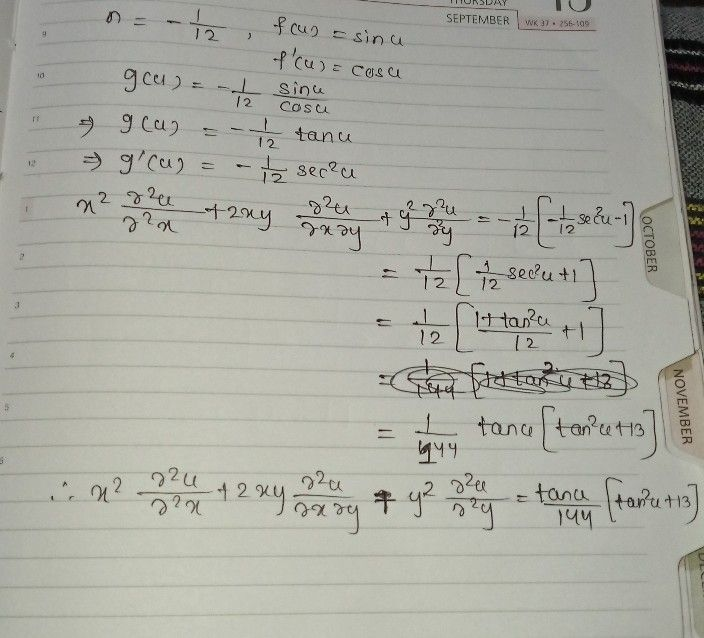Symbol
Problem$1/3$ $Ifa=sin^{-1}\left(\dfrac {x} {112}$ $\dfrac {x^{1/3}+y^{1/3}} {x^{1/2}+y^{1/2}}\right)^{1/2}$ prove that $x^{2}\dfrac {0^{2}} {Ox^{2}}+2xy\dfrac {θ^{2}n} {0x0y}+y^{2}\dfrac {θ^{2}x} {θy^{2}}=\dfrac {tant} {144}\left(13+tan^{2}$ $\right)$
10th-13th grade
Other
Search count: 107
SolutionQanda teacher - priyamamunStudent
hey can i asked 1 more question?. if u r not busy..